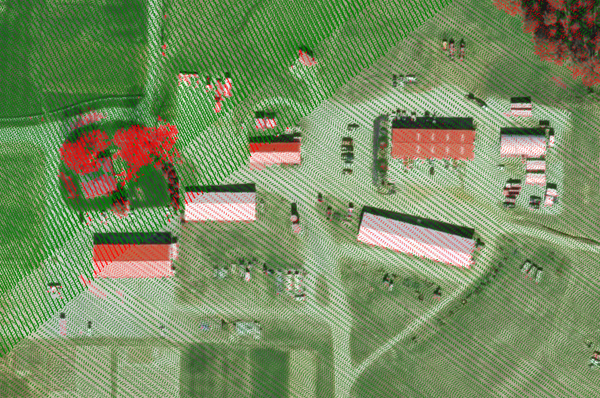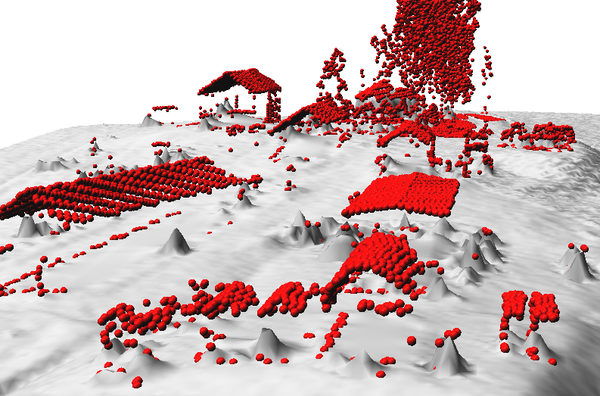## NAME

v.lidar.edgedetection - Detects the object's edges from a LIDAR data set.

## KEYWORDS

vector, LIDAR, edges

## SYNOPSIS

v.lidar.edgedetection
v.lidar.edgedetection --help
v.lidar.edgedetection [-e] input=name output=name [ew_step=float] [ns_step=float] [lambda_g=float] [tgh=float] [tgl=float] [theta_g=float] [lambda_r=float] [--overwrite] [--help] [--verbose] [--quiet] [--ui]

### Flags:

-e
Estimate point density and distance and quit
Estimate point density and distance in map units for the input vector points within the current region extents and quit
--overwrite
Allow output files to overwrite existing files
--help
Print usage summary
--verbose
Verbose module output
--quiet
Quiet module output
--ui
Force launching GUI dialog

### Parameters:

input=name [required]
Name of input vector map
Or data source for direct OGR access
output=name [required]
Name for output vector map
ew_step=float
Length of each spline step in the east-west direction
Default: 4 * east-west resolution
ns_step=float
Length of each spline step in the north-south direction
Default: 4 * north-south resolution
lambda_g=float
Default: 0.01
tgh=float
High gradient threshold for edge classification
Default: 6
tgl=float
Low gradient threshold for edge classification
Default: 3
theta_g=float
Angle range for same direction detection
Default: 0.26
lambda_r=float
Regularization weight in residual evaluation
Default: 2

## DESCRIPTION

v.lidar.edgedetection is the first of three steps to filter LiDAR data. The filter aims to recognize and extract attached and detached object (such as buildings, bridges, power lines, trees, etc.) in order to create a Digital Terrain Model.

In particular, this module detects the edge of each single feature over the terrain surface of a LIDAR point surface. First of all, a bilinear spline interpolation with a Tychonov regularization parameter is performed. The gradient is minimized and the low Tychonov regularization parameter brings the interpolated functions as close as possible to the observations. Bicubic spline interpolation with Tychonov regularization is then performed. However, now the curvature is minimized and the regularization parameter is set to a high value. For each point, an interpolated value is computed from the bicubic surface and an interpolated gradient is computed from the bilinear surface. At each point the gradient magnitude and the direction of the edge vector are calculated, and the residual between interpolated and observed values is computed. Two thresholds are defined on the gradient, a high threshold tgh and a low one tgl. For each point, if the gradient magnitude is greater than or equal to the high threshold and its residual is greater than or equal to zero, it is labeled as an EDGE point. Similarly a point is labeled as being an EDGE point if the gradient magnitude is greater than or equal to the low threshold, its residual is greater than or equal to zero, and the gradient to two of eight neighboring points is greater than the high threshold. Other points are classified as TERRAIN.

The length (in mapping units) of each spline step is defined by ew_step for the east-west direction and ns_step for the north-south direction.

The output will be a vector map in which points has been classified as TERRAIN, EDGE or UNKNOWN. This vector map should be the input of v.lidar.growing module.

## NOTES

In this module, an external table will be created which will be useful for the next module of the procedure of LiDAR data filtering. In this table the interpolated height values of each point will be recorded. Also points in the output vector map will be classified as:
```TERRAIN (cat = 1, layer = 1)
EDGE (cat = 2, layer = 1)
UNKNOWN (cat = 3, layer = 1)
```
The final result of the whole procedure (v.lidar.edgedetection, v.lidar.growing, v.lidar.correction) will be a point classification in four categories:
```TERRAIN SINGLE PULSE (cat = 1, layer = 2)
TERRAIN DOUBLE PULSE (cat = 2, layer = 2)
OBJECT SINGLE PULSE (cat = 3, layer = 2)
OBJECT DOUBLE PULSE (cat = 4, layer = 2)
```

## EXAMPLES

### Basic edge detection

```# last return points
v.lidar.edgedetection input=vector_last output=edge ew_step=8 ns_step=8 lambda_g=0.5
```

### Complete workflow

```# region settings (using an existing raster)
g.region raster=elev_lid792_1m

# import
v.in.lidar -tr input=points.las output=points
v.in.lidar -tr input=points.las output=points_first return_filter=first

# detection
v.lidar.edgedetection input=points output=edge ew_step=8 ns_step=8 lambda_g=0.5
v.lidar.growing input=edge output=growing first=points_first
v.lidar.correction input=growing output=correction terrain=only_terrain

# visualization of selected points
# zoom somewhere first, to make it faster
d.rast map=orthophoto
d.vect map=correction layer=2 cats=2,3,4 color=red size=0.25
d.vect map=correction layer=2 cats=1 color=0:128:0 size=0.5

# interpolation (this may take some time)
v.surf.rst input=only_terrain elevation=terrain

# get object points for 3D visualization
v.extract input=correction layer=2 cats=2,3,4 output=objects
```Figure 1: Example output from complete workflow (red: objects, green: terrain)Figure 2: 3D visualization of filtered object points (red) and terrain created from terrain points (gray)

## REFERENCES

• Antolin, R. et al., 2006. Digital terrain models determination by LiDAR technology: Po basin experimentation. Bolletino di Geodesia e Scienze Affini, anno LXV, n. 2, pp. 69-89.
• Brovelli M. A., Cannata M., Longoni U.M., 2004. LIDAR Data Filtering and DTM Interpolation Within GRASS, Transactions in GIS, April 2004, vol. 8, iss. 2, pp. 155-174(20), Blackwell Publishing Ltd.
• Brovelli M. A., Cannata M., 2004. Digital Terrain model reconstruction in urban areas from airborne laser scanning data: the method and an example for Pavia (Northern Italy). Computers and Geosciences 30 (2004) pp.325-331
• Brovelli M. A. and Longoni U.M., 2003. Software per il filtraggio di dati LIDAR, Rivista dell'Agenzia del Territorio, n. 3-2003, pp. 11-22 (ISSN 1593-2192).
• Brovelli M. A., Cannata M. and Longoni U.M., 2002. DTM LIDAR in area urbana, Bollettino SIFET N.2, pp. 7-26.
• Performances of the filter can be seen in the ISPRS WG III/3 Comparison of Filters report by Sithole, G. and Vosselman, G., 2003.

v.lidar.growing, v.lidar.correction, v.surf.bspline, v.surf.rst, v.in.lidar, v.in.ascii

## AUTHORS

Original version of program in GRASS 5.4:
Maria Antonia Brovelli, Massimiliano Cannata, Ulisse Longoni and Mirko Reguzzoni

Update for GRASS 6.X:
Roberto Antolin and Gonzalo Moreno

## SOURCE CODE

Available at: v.lidar.edgedetection source code (history)

Latest change: Thursday Feb 03 11:10:06 2022 in commit: 547ff44e6aecfb4c9cbf6a4717fc14e521bec0be

© 2003-2022 GRASS Development Team, GRASS GIS 8.2.0 Reference Manual